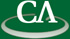## Sparse Polynomial Interpolation.

### 11:30am, Tuesday September 2nd, 2014, K9509.

```Abstract

Polynomial interpolation is the problem of reconstructing a polynomial from a
set of its evaluations or modular images. Polynomial interpolation is a
fundamental tool of computer algebra and has applications to power series,
polynomial, and integer arithmetic.

We consider the problem of interpolating a polynomial that is sparse, i.e., a
polynomial with only a small number of nonzero terms, such that the true "size"
of the polynomial is much smaller than its degree.  In this talk we discuss
recently-developed randomized techniques for interpolating sparse polynomials,
with different methods to handle the cases of univariate, bivariate, and
higher-dimensional multivariate polynomials.  This is joint work with Mark
Giesbrecht and Daniel Roche.

```